Latest Banking jobs   »   Quantitative Aptitude Quiz For SBI Clerk...

# Quantitative Aptitude Quiz For SBI Clerk Mains Exam 2021- 21st August

Directions (1-5): Given pie chart show the population distribution of five cities and 2nd pie chart show the male population distribution of these cities.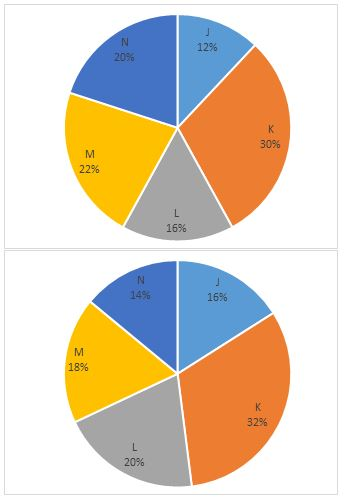Note: Ratio of total population of five cities to total male population of these cities is 3 : 2.

Q1. If population of city K is 1,80,000 then find the female population of city L.
(a) 20000
(b) 18000
(c) 22000
(d) 14000
(e) 16000

Q2. What is the ratio of female population of city M to the female population of city J.
(a) 2 : 15
(b) 15 : 2
(c) 7 : 15
(d) 3 : 16
(e) 4 : 15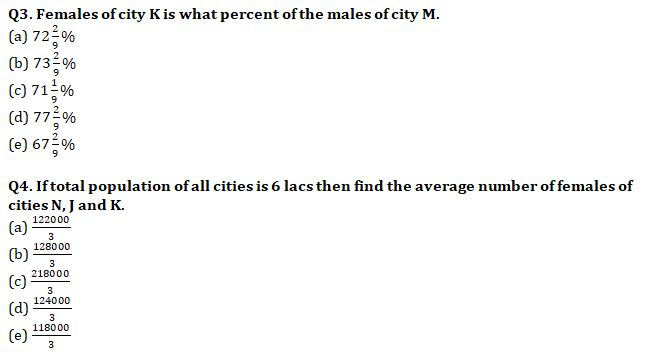Q5. If male population of city L is 1 lakh then find that female population of city M is what percent of the male population of city K.
(a) 43.875%
(b) 47%
(c) 46.875%
(d) 46.125%
(e) 47.625%

Directions (6-10): The given table show the S.I. and C.I. rate per annum given by five lenders.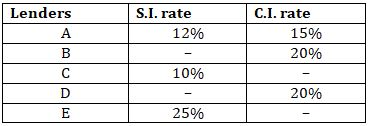Note: Some values are missing, you must calculate these values according to question.

Q6. Lender A, lends some amount at S.I. for 3 years and another amount, which is 1500 more than the previous one, at C.I. for 2 years. Both sum earns equal amount. Find the amount lends at C.I.
(a) 12900
(b) 13500
(c) 15000
(d) 14400
(e) 11500

Q7. Lender C lends a sum for 2 years at S.I. then after 2 year he re-lend the total amount for 2 years at C.I. and earn a total profit which is 45.2% of sum invest by him in S.I. Find the rate of C.I.
(a) 10%
(b) 11%
(c) 12%
(d) 20%
(e) 15%

Q8. If D lend money in S.I. for 8 year he earns double Interest as he earns on same money in 2 years at C.I. then find the interest he earns on 1500 at S.I. in 3 year.
(a) 500 Rs.
(b) 360 Rs.
(c) 900 Rs.
(d) 495 Rs.
(e) 600 Rs.

Q9. Interest earn by E in 2 years at C.I. is 25% more than the interest earns by him in 4 years at S.I. on same amount. What is the ratio of amount occurred when same sum is lend by D and E for 3 years at C.I.
(a) 36 : 125
(b) 64 : 113
(c) 64 : 125
(d) 75 : 113
(e) 216 : 125

Q10. When B lends same sum on S.I. and C.I. each, he earn interest in ratio of 3 : 2 in which he lend amount at S.I. for 3 years and at C.I. for 2 years. If difference between total interest earn in S.I. and C.I. is 2640 Rs. then find rate at S.I. lends by B ?
(a) 20%
(b) 22%
(c) 24%
(d) 10%
(e) 25%

Directions (11-15): In each of these questions, two equations (I) and (II) are given. You have to solve both the equations and give answer
(a) if x>y
(b) if x≥y
(c) if x<y
(d) if x ≤y
(e) if x = y or No relation can be established between x and y.

Q11. (i) x² = (23)² – 5 × 77
(ii) 2y² + 51y + 324 = 0

Q12. (i) 3x² – 58x + 280 = 0
(ii) 3y² – 67y + 374 = 0

Q13. (i) 5x² = 5x + 176/5
(ii) 25y² – 55y + 18 = 0

Q14. (i) x² – 41/20x + 1 = 0
(ii) 16y² – 22y + 7 = 0

Q15. (i) 2x – y = 31/15
(ii) 3x + 5y = 20

Solutions: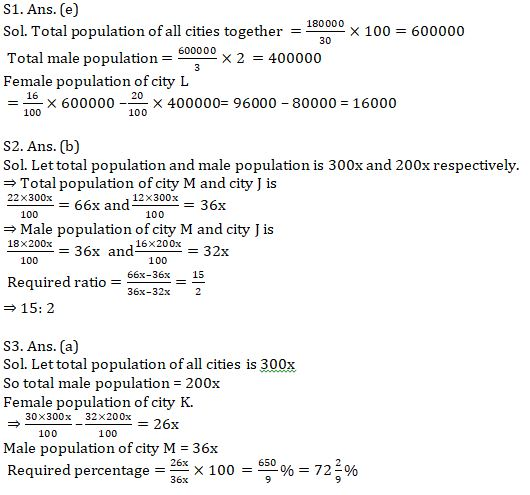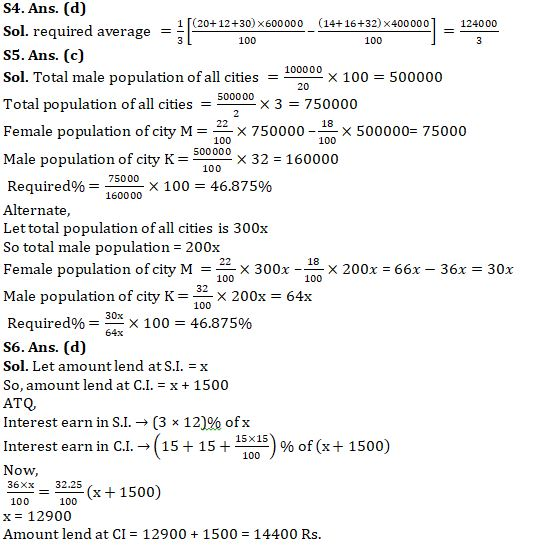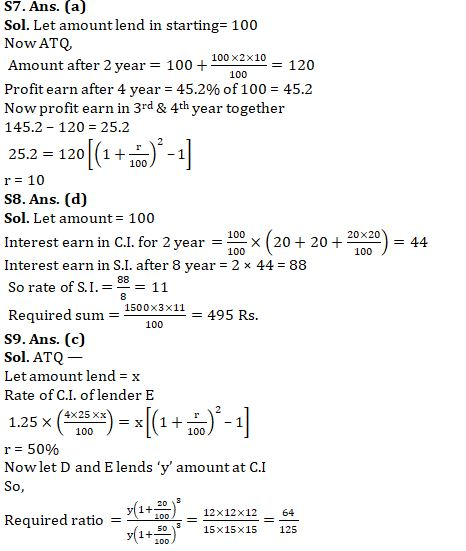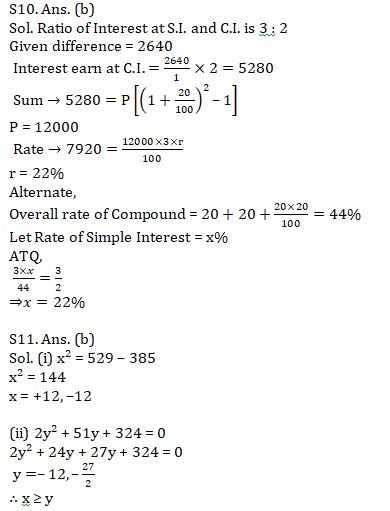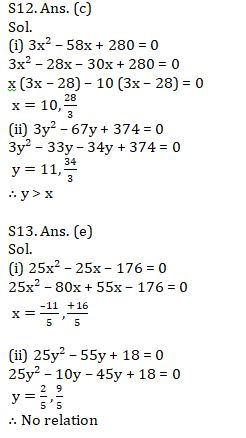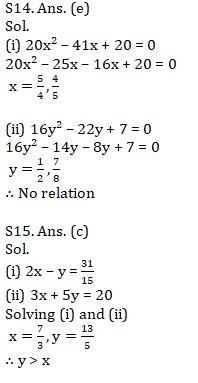#### Congratulations!